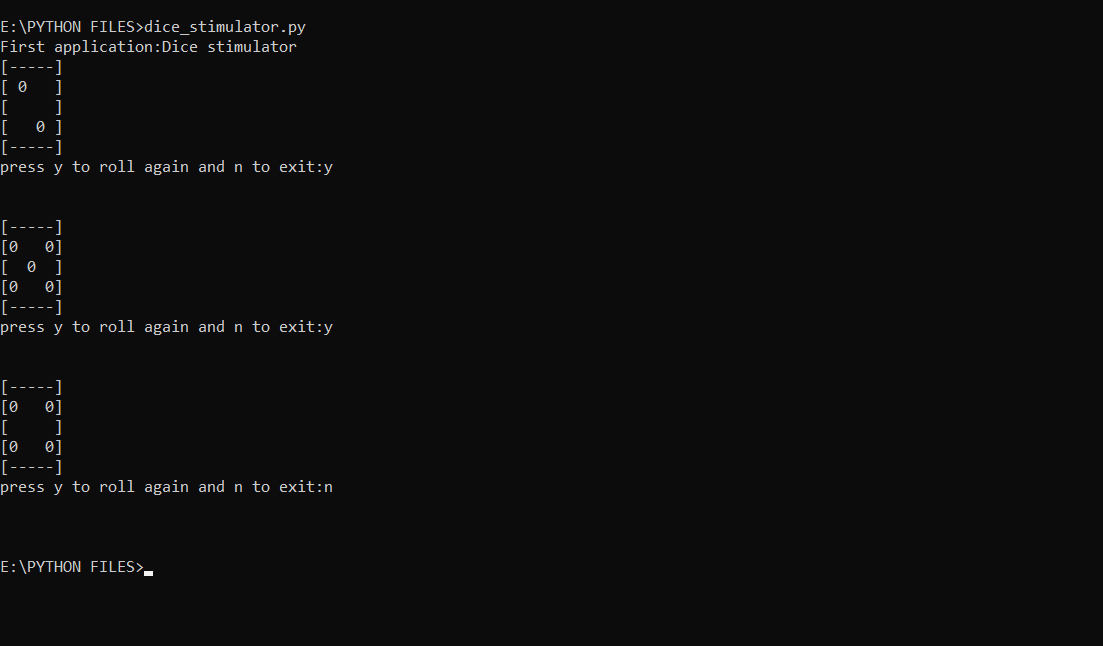# Dice Rolling Simulator using Python-random

In this article we will create a classic rolling dice simulator with the help of basic Python knowledge. Here we will be using the random module since we randomize the dice simulator for random outputs.

Function used:

1) random.randint(): This function generates a random number in the given range.

Below is the implementation.

 `import` `random ` ` `  ` `  `x ``=` `"y"` `  `  `while` `x ``=``=` `"y"``: ` `     `  `    ``# Gnenerates a random number ` `    ``# between 1 and 6 (including ` `    ``# both 1 and 6) ` `    ``no ``=` `random.randint(``1``,``6``) ` `     `  `    ``if` `no ``=``=` `1``: ` `        ``print``(``"[-----]"``) ` `        ``print``(``"[     ]"``) ` `        ``print``(``"[  0  ]"``) ` `        ``print``(``"[     ]"``) ` `        ``print``(``"[-----]"``) ` `    ``if` `no ``=``=` `2``: ` `        ``print``(``"[-----]"``) ` `        ``print``(``"[ 0   ]"``) ` `        ``print``(``"[     ]"``) ` `        ``print``(``"[   0 ]"``) ` `        ``print``(``"[-----]"``) ` `    ``if` `no ``=``=` `3``: ` `        ``print``(``"[-----]"``) ` `        ``print``(``"[     ]"``) ` `        ``print``(``"[0 0 0]"``) ` `        ``print``(``"[     ]"``) ` `        ``print``(``"[-----]"``) ` `    ``if` `no ``=``=` `4``: ` `        ``print``(``"[-----]"``) ` `        ``print``(``"[0   0]"``) ` `        ``print``(``"[     ]"``) ` `        ``print``(``"[0   0]"``) ` `        ``print``(``"[-----]"``) ` `    ``if` `no ``=``=` `5``: ` `        ``print``(``"[-----]"``) ` `        ``print``(``"[0   0]"``) ` `        ``print``(``"[  0  ]"``) ` `        ``print``(``"[0   0]"``) ` `        ``print``(``"[-----]"``) ` `    ``if` `no ``=``=` `6``: ` `        ``print``(``"[-----]"``) ` `        ``print``(``"[0 0 0]"``) ` `        ``print``(``"[     ]"``) ` `        ``print``(``"[0 0 0]"``) ` `        ``print``(``"[-----]"``) ` `         `  `    ``x``=``input``(``"press y to roll again and n to exit:"``) ` `    ``print``(``"\n"``) `

Output:My Personal Notes arrow_drop_upCheck out this Author's contributed articles.

If you like GeeksforGeeks and would like to contribute, you can also write an article using contribute.geeksforgeeks.org or mail your article to contribute@geeksforgeeks.org. See your article appearing on the GeeksforGeeks main page and help other Geeks.

Please Improve this article if you find anything incorrect by clicking on the "Improve Article" button below.

Article Tags :

Be the First to upvote.

Please write to us at contribute@geeksforgeeks.org to report any issue with the above content.